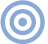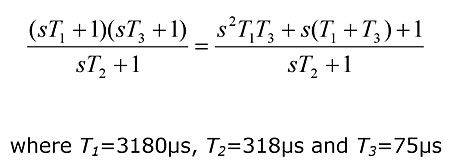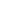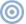# Features

## Cut and Thrust: RIAA LP Equalization The Neumann 4th pole (sic)

Sidebar: The Neumann 4th pole (sic)

Using the phrase "4th pole" to describe the addition of a further time constant to curtail the RIAA record EQ at ultrasonic frequencies is a misnomer. Talk of poles takes us into what will be unfamiliar territory for many Stereophile readers, so a brief—and necessarily simplified—explanation is given here.

Filter circuits are described by a mathematical equation called a transfer function, which in general form is a ratio of two polynomials (ie, power series) in a variable denoted s (s=jw, where w is angular frequency (2PIf) and j is the square root of –1). The RIAA preemphasis curve, for example, has the transfer functionThis equation describes the ratio of the output voltage to the input voltage and can be solved to determine the circuit's amplitude vs frequency and phase vs frequency responses.

Significant features of these responses occur at frequencies corresponding to the roots of the two polynomials, which are referred to as zeros and poles. Because the transfer function of the RIAA preemphasis curve has an s2 term in the numerator, it has two zeros (at 50.05 and 2122Hz); and because the highest order of s in the denominator is 1, it has a single pole (at 500.5Hz). As the RIAA deemphasis curve is the inverse of the preemphasis curve, its poles and zeros are flipped: it has two poles (at 50.05 and 2122Hz) and one zero (at 500.5Hz). Applying the IEC 7950µs replay time constant adds a further pole and zero, the latter at 0Hz.

We can see from this that the term "4th pole" is inaccurate. The RIAA record characteristic has only one pole, so adding another at 3.18µs would increase the pole count to two. On the replay side, correcting for this additional pole means adding a further zero, so that even including the additional IEC low-frequency time constant, the pole count remains three.—Keith HowardARTICLE CONTENTSX